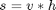# Separable convolution: Part 2

Back in October I introduced the concept of filter separability. A two-dimensional filter s is said to be separable if it can be written as the convolution of two one-dimensional filters v and h:I said then that "next time" I would explain how to determine whether a given filter is separable. Well, I guess I got side-tracked, but I'm back on topic now.

This question gave me one of earliest opportunities at The MathWorks to wander down to company co-founder Cleve's office and ask for advice. I asked, "How can I determine if a matrix is an outer product of two vectors?" Cleve was very helpful, as he always is, although I was a little embarrassed afterward that I hadn't figured it out myself. "Go look at the rank function," Cleve told me.

Of course. If a matrix is an outer product of two vectors, its rank is 1. Here are the key lines of code in rank:

dbtype 15:19 rank
15    s = svd(A);
16    if nargin==1
17       tol = max(size(A)') * eps(max(s));
18    end
19    r = sum(s > tol);



So the test is this: The rank of A is the number of nonzero singular values of A, with some numerical tolerance based on eps and the size of A.

Let's try it with a few common filters.

An averaging filter should be obvious:

averaging = ones(5,5) / 25;
rank(averaging)
ans =

1



The Sobel kernel:

sobel = [-1 0 1; -2 0 2; -1 0 1];
rank(sobel)
ans =

1



The two-dimensional Gaussian is the only radially symmetric function that is also separable:

gaussian = fspecial('gaussian');
rank(gaussian)
ans =

1



A disk is not separable:

disk = fspecial('disk');
rank(disk)
ans =

5



So how can we determine the outer product vectors? The answer is to go back to the svd function. Here's a snippet from the doc: [U,S,V] = svd(X) produces a diagonal matrix S of the same dimension as X, with nonnegative diagonal elements in decreasing order, and unitary matrices U and V so that X = U*S*V'.

A rank 1 matrix has only one nonzero singular value, so U*S*V' becomes U(:,1) * S(1,1) * V(:,1)'. This is basically the outer product we were seeking. Therefore, we want the first columns of U and V. (We have to remember also to use the nonzero singular value as a scale factor.)

Let's try this with the Gaussian filter.

[U,S,V] = svd(gaussian)
U =

-0.1329    0.9581   -0.2537
-0.9822   -0.1617   -0.0959
-0.1329    0.2364    0.9625

S =

0.6420         0         0
0    0.0000         0
0         0    0.0000

V =

-0.1329   -0.6945   -0.7071
-0.9822    0.1880    0.0000
-0.1329   -0.6945    0.7071



Now get the horizontal and vertical vectors from the first columns of U and V.

v = U(:,1) * sqrt(S(1,1))
v =

-0.1065
-0.7870
-0.1065


h = V(:,1)' * sqrt(S(1,1))
h =

-0.1065   -0.7870   -0.1065



I have chosen, somewhat arbitrarily to split the scale factor, S(1,1), "equally" between v and h.

Now check to make sure this works:

gaussian - v*h
ans =

1.0e-015 *

-0.0243   -0.1527   -0.0243
-0.0139   -0.1110   -0.0139
-0.0035         0   -0.0035



Except for normal floating-point roundoff differences, gaussian and v*h are equal.

You can find code similar to this in the MATLAB function filter2, as well as in the Image Processing Toolbox function imfilter.

Published with MATLAB® 7.3

|

### Comments

To leave a comment, please click here to sign in to your MathWorks Account or create a new one.